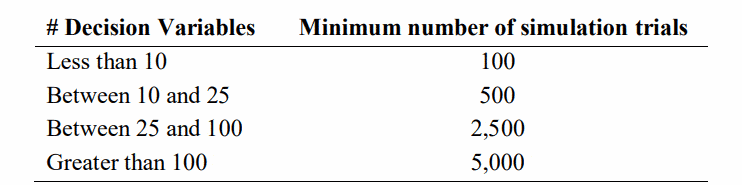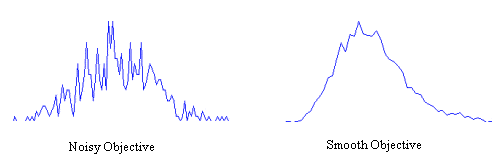# How Many Simulation Trials Should I Run? Factors that Impact OptQuest Search PerformanceThe industry leading OptQuest simulation optimization engine from OptTek is integrated with AnyLogic to further enhance analysis and decision making with simulation modeling.

OptQuest features sophisticated analysis techniques and powerful algorithms that are unique to the optimizer. They are used across verticals by commercial, military, and governmental organizations, enabling automatic discovery of optimal parameters when solving problems with simulation.

The recent release of AnyLogic 8.5 included the latest OptQuest optimizer. Read on and learn from Dr. Ben Thengvall, OptTek Optimization Specialist, about how to quickly determine optimal parameters for your simulation models with OptQuest.

Without known bounds, a heuristic method cannot guarantee that it will find the optimal solution to a simulation optimization problem unless it enumerates all possibilities. However, this brute force approach of exploring all possibilities is often not practical due to limitations on time and computing resources. OptQuest is designed to find the best solution or very good solutions to complex problems with a minimum number of simulation evaluations.

A question that is commonly asked by OptQuest users is: “How many simulation trials do I need to run to find the best, or a very good, solution?” There is no simple answer to this question, because the answer depends on many factors. However, the following is a list of relevant factors that directly affect search performance (how quickly OptQuest will find the best solution). Each factor is explained in the following sections:

### Number of Decision Variables

The number of decision variables greatly affects how many simulation trials you should allow OptQuest to perform to find good solutions. OptQuest has no physical limit on the number of decision variables that can be used in any given problem. However, the larger the number of decision variables there are in a problem, the more simulation trials that should be executed to find the best solutions. General guidelines for the minimum number of simulation trials required for a given number of decision variables in a problem are:Sometimes, rather than running an optimization with a very large number of variables, the number of variables can be reduced to focus the search on the more important decision variables. This reduction can be facilitated through variable sensitivity analysis, piecewise optimization, or selecting rulesets of preconfigured parameters.

### Initial Values

Suggested starting solutions can be passed to OptQuest to initiate the search process. If suggested solutions are provided, OptQuest will evaluate these first, and use them to seed the optimization search. Good starting solutions can significantly improve overall optimization performance for large problems because OptQuest does not have to spend additional time finding these good areas of the solution space.

### Decision Variable Bounds

Performance can be significantly improved by selecting meaningful bounds for the decision variables. Suppose, for example, that the bounds for three decision variables (X, Y, and Z) are:

• 0 <= X <= 100
• 0 <= Y <= 100
• 0 <= Z <= 100

If these bounds are valid, but the analyst knows that a smaller range of values is interesting for the current study, then more restrictive bounds should be used, for example:

• 15 <= X <= 65
• 0 <= Y <= 16.667
• 10 <= Z <= 80

These tighter bounds result in a more efficient search for the optimal values of the decision variables since they reduce the possible values that OptQuest must consider.

### Complexity of the Objective

A complex objective has a highly nonlinear surface with many local minimum and maximum points or is noncontiguous at one or more points.

OptQuest is designed to find global optimal solutions for all types of objectives, especially complex objectives. However, for more complex objectives, generally it is required to run more simulations to find high-quality solutions.

### Number of Objectives

Almost all practical problems have more than one objective. Commonly there are at least two objectives of interest, one a measure of cost, and the second a measure of performance. These two objectives are almost always inversely related, or competing, and the various optimal tradeoffs between cost and performance are desired for a decision maker to evaluate.

More simulation trials are needed when optimizing multiple objectives. OptQuest will spend some time focusing the search on each objective and then it will focus on filling in the gaps on the Pareto frontier. As a rough rule, multiply the number of suggested simulation trials in Table 1 above by 5 when solving multi-objective problems. For example, allowing OptQuest at least 500 simulation trials is recommended for a problem with less than 10 decision variables.

OptQuest allows any number of objectives, but it is often best to optimize with two objectives, because it makes interpreting the results more straightforward. If there are more than two relevant objectives, one recommended approach is to select the two most important objectives and optimize them first. Then add the objectives already optimized as constraints restricted to be within some high percentage of their optimal values and run the optimization again with another objective selected.

### Constraints

Constraints can be used to restrict the values of decision variables (model parameters) by defining relationships among the decision variables; constraints can also restrict the value of output variables (performance measures).

If a linear constraint is defined using only decision variables, OptQuest can eliminate decision variable values that are constraint-infeasible before it runs the simulation. Therefore, limiting the optimization by defining constraints on decision variables generally improves search performance.

If a constraint contains a performance measure, a simulation must be run to determine whether the suggested solution satisfies the constraint.

### Number of Replications per Simulation Trial

When OptQuest runs an optimization, it runs a simulation to evaluate each set of decision variable (model parameter) values. Therefore, the quality of the optimization results depends on the number of simulation trials (iterations) and the number of replications per simulation trial.

For a set period of time, the number of replications per simulation trial is inversely related to the number of simulation trials that can be completed; as one increases, the other decreases. Decreasing the number of replications will increase the number of simulation trials executed in a given amount of time.

The more simulation trials OptQuest can run, the more sets of values it can evaluate, and the more likely OptQuest is to find a solution close to the optimal solution. Therefore, there is a trade-off between statistical precision through more replications and solution quality with more simulation trials.

### Simulation Accuracy

There are two factors that affect simulation accuracy:

• Number of replications per simulation
• Noisiness of the objective

For sufficient accuracy, the number of replications per simulation must be set to the minimum number necessary to obtain a reliable estimate of the objective function being optimized; this minimum number is typically found with empirical testing.

Noisiness can also affect the accuracy of OptQuest results.The objective on the left in Figure 1 has significant amounts of noise caused by the probability distributions used to model the problem’s uncertainty. For these types of objectives, OptQuest might have trouble discerning the minimum or maximum value. Noisy functions can be detected by watching for best solutions that seem to "bounce around" from one set of values to completely different sets of values. To help solve this problem, increase the number of replications per simulation. On the right in Figure 1, the objective appears smooth due to the relative certainty in the model assumptions. In these cases, OptQuest should quickly converge to the best solution.

For a deeper dive into using OptQuest in AnyLogic, you can find documentation in AnyLogic help and in our Optimization Experiment how-to video:

You can find examples of OptQuest in use in our case studies, papers, and conference videos, as well as further information about OptQuest from OptTek: